# GSEB Solutions Class 12 Physics Chapter 4 Moving Charges and Magnetism

Gujarat Board GSEB Textbook Solutions Class 12 Physics Chapter 4 Moving Charges and Magnetism Textbook Questions and Answers, Additional Important Questions, Notes Pdf.

## Gujarat Board Textbook Solutions Class 12 Physics Chapter 4 Moving Charges and Magnetism

### GSEB Class 12 Physics Moving Charges and Magnetism Text Book Questions and AnswersQuestion 1.
A circular coil of wire consisting of 100 turns, each of radius 8.0 cm carries a current of 0.40 A. What is the magnitude of the magnetic field B at the centre of the coil?
Solution:
n = 100, I = 0.40 A, r = 8.0 cm = 8.0 x 10-2 m
B = $$\frac{\mu_{0} \mathrm{nI}}{2 \mathrm{r}}$$
= $$\frac{4 \pi \times 10^{-7} \times 100 \times 0.4}{2 \times 8 \times 10^{-2}}$$
= π x 10-4 T

Question 2.
A long straight wire carries a current of 35 A. What is the magnitude of the field B at a point 20 cm from the w ire?
Solution:
I = 35 A, r = 20 cm = 20 x 10-2 m
B = $$\frac{\mu_{0} I}{2 \pi r}$$
= $$\frac{4 \pi \times 10^{-7} \times 35}{2 \pi \times 20 \times 10^{-2}}$$
= 3.5 x 10-5 TQuestion 3.
A long straight wire in the horizontal plane carries a current of 50 A in north to south direction. Give the magnitude and direction of B at a point 2.5 m east of the wire.
Solution:
I = 50 A, r = 2.5 m
B = $$\frac{\mu_{0} I}{2 \pi r}$$
= $$\frac{4 \pi \times 10^{-7} \times 50}{2 \pi \times 2.5}$$
= 4 x 10-6 T upwards. (due to the wire alone)

Question 4.
A horizontal overhead power line carries a current of 90 A in east to west direction. What is the magnitude and direction of the magnetic field due to the current 1.5 m below the line?
Solution:
I = 90 A, r = 1.5 m
B = $$\frac{\mu_{0} I}{2 \pi r}$$
= $$\frac{4 \pi \times 10^{-7} \times 90}{2 \pi \times 1.5}$$
= 1.2 x 10-5 T (South)Question 5.
What is the magnitude of magnetic force per unit length on a wire carrying a current of 8 A and making an angle of 30° with the direction of a uniform magnetic field of 0.15T?
Solution:
I = 8A, B = 0.15 T and θ = 30°
F = BI1 sin θ
Therefore, force per unit length on the wire,
F = 0.15 × 8 x 1 x sin30°
= 0.15 x 8 x 1 x 0.5
= 0.6 Nm-1

Question 6.
A 3.0 cm wire carrying a current of 10 A is placed inside a solenoid perpendicular to its axis. The magnetic field inside the solenoid is given to be 0.27 T. What is the magnetic force on the wire?
Solution:
I = 3.0 cm = 3.0 x 10-2 m
I = 10 A
B = 0.27 T
F = BIl = 0.27 x 10 x 3 x 10-2
= 8.1 x 10-2 N

Question 7.
Two long and parallel straight wires A and B carrying currents of 8.0 A and 5.0 A in the same direction are separated by a distance of 4.0 cm. Estimate the force on a 10 cm section of wire A.
Solution:
I1 = 8.0 A
I2 = 5.0 A
r = 4.0 cm = 4.0 x 10-2 m
l = 10 cm = 0.1 m
F = $$\frac{2 \times 10^{-7} \mathrm{I}_{1} \mathrm{I}_{2} l}{\mathrm{r}}$$
= $$\frac{2 \times 10^{-7} \times 8 \times 5 \times 0.1}{4 \times 10^{-2}}$$
= 2 x 10-5 N (towards B)Question 8.
A closely wound solenoid 80 cm long has 5 layers of windings of 400 turns each. The diameter of the solenoid is 1.8 cm. If the current carried is 8.0 A, estimate the magnitude of B inside the solenoid near its centre.
Solution:
l = 80 cm = 80 x 10-2 m
p = 5
N = 400
n = $$\frac { N }{ l }$$
= $$\frac{400}{80 \times 10^{-2}}$$
= 500
For one turn, B = μ0nl
B = 4π x 10-7 x 500 x 8
For p turns = pB
= 5 x 4π x 10-7 x 500 x 8
= 8π x 10-3 T

Question 9.
A square coil of side 10 cm consists of 20 turns and carries a current of 12 A. The coil is suspended vertically and the normal to the plane of the coil makes an angle of 30° with the direction of a uniform horizontal magnetic field of magnitude 0.80 T. What is the magnitude of torque experienced by the coil?
Solution:
I = 12A
n = 20
B = 0.80 T
θ =30°
A = 10 x 10= 100cm2
= 100 x 104m2
τ = n BIA sin θ
= 2d x 0.80 x l00 x l0-4 x l2 x sin30°
= 0.96 Nm.Question 10.
Two moving coil meters, M1 and M2 have the following particulars:
R1 = 10 Ω, N1 = 30, A1 = 3.6 x 10-3 m2, B1 = 0.25 T R2 = 14 Ω, N2 = 42, A2 = 1.8 x 10-3 m2, B2 = 0.50 T
(The spring constants are identical for the two meters).
Determine the ratio of (a) current sensitivity and (b) voltage sensitivity of M2 and M1.
Solution: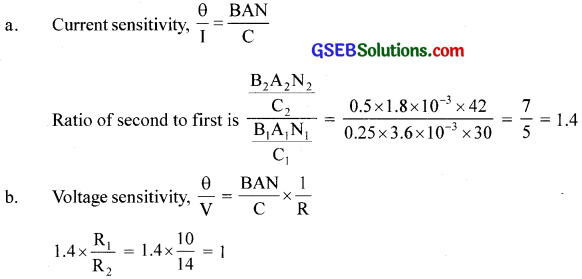Question 11.
In a chamber, a uniform magnetic field of 6.5G (1G = 10-4 T) is maintained. An electron is shot into the field with a speed of 4.8 x 106 ms-1 normal to the field. Explain why the path of the electron is a circle. Determine the radius of the circular orbit. (e = 1.6 x 10-19 C, me = 9.1 x 10-31 kg)
Solution:
B = 6.5 x 10-4 T, v = 4.8 x 106ms-1
Bqv = $$\frac{\mathrm{mv}^{2}}{\mathrm{r}}$$
r = $$\frac { mv }{ qB }$$
= $$\frac{9.1 \times 10^{-31} \times 4.8 \times 10^{6}}{1.6 \times 10^{-19} \times 6.5 \times 10^{-4}}$$
= 4.2 x 10-2m
= 4.2 cm

Question 12.
In Exercise. 11 obtain the frequency of revolution of the electron in its circular orbit. Does the answer depend on the speed of the electron? Explain.
Solution:
Frequency in Exercise 11,
υ = $$\frac { qB }{ 2πm }$$
= $$\frac{1.6 \times 10^{-19} \times 6.5 \times 10^{-4}}{2 \times \pi \times 9.1 \times 10^{-31}}$$
= 18 MHzQuestion 13.
(a) A circular coil of 30 turns and radius 8.0 cm carrying a current of 6.0 A is suspended vertically in a uniform horizontal magnetic field of magnitude 1.0 T. The field lines make an angle of 60° with the normal of the coil. Calculate the magnitude of the counter-torque that must be applied to prevent the coil from turning.
(b) Would your answer change, if the circular coil in (a) were replaced by a planar coil of some irregular shape that encloses the same area? (All other particulars are also unaltered.)
Solution:
(a) N = 30, r = 8 x 10”2m, I = 6A, B = 1T, θ = 60°
τ = BANI sin 60° = 1 x π x (8 x 10-2)2 x 30 x 6 x $$\frac { \sqrt { 3 } }{ 2 }$$
= 3.1 Nm

(b) No change.
∵ $$\vec { \tau }$$ = NI($$\vec { A }$$ x $$\vec { B }$$)

Question 14.
Two concentric circular coils X and Y of radii 16 cm and 10 cm, respectively, lie in the same vertical plane containing the north to south direction. Coil X has 20 turns and carries a current of 16 A; coil Y has 25 turns and carries a current of 18 A. The sense of the current in X is anticlockwise, and clockwise in Y, for an observer looking at the coils facing west. Give the magnitude and direction of the net magnetic field due to the coils at their centre.
Solution: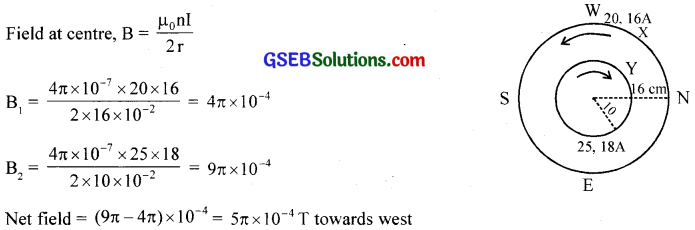Question 15.
For a circular coil of radius R and N turns carrying current I, the magnitude of the magnetic field at a point on its axis at a distance x from its centre is given by,
B = $$\frac{\mu_{0} I R^{2} N}{2\left(x^{2}+R^{2}\right)^{3 / 2}}$$
(a) Show that this reduces to the familiar result for the field at the centre of the coil.
(b) Consider two parallel co-axial circular coils of equal radius R, and number of turns N, carrying equal currents in the same direction, and separated by a distance R. Show that the field on the axis around the mid-point between the coils is uniform over a distance that is small as compared to R, and is given by,
B = 0.72 $$\frac{\mu_{0} \mathrm{NI}}{\mathrm{R}}$$, approximately.
[Such an arrangement to produce a nearly uniform magnetic field over a small region is known as Helmholtz coils.]
Solution: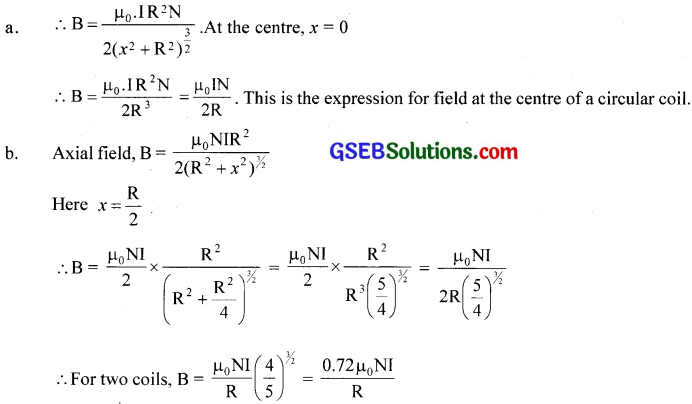Question 16.
A toroid has a core (non-ferromagnetic) of inner radius 25 cm and outer radius 26 cm, around which 3500 turns of a wire are wound. If the current in the wire is 11 A, what is the magnetic field
(i) outside the toroid
(ii) inside the core of the toroid
(iii) in the empty space surrounded by the toroid.
Solution:
(i) Zero
(ii) B = μ0NI = $$\frac{4 \pi \times 10^{-7} \times 3500 \times 11}{2 \pi \times 25.5 \times 10^{-2}}$$
(iii) ZeroQuestion 17.
(a) A magnetic field that varies in magnitude from point to point but has a constant direction (east to west) is set up in a chamber. A charged particle enters the chamber and travels undeflected along a straight path with constant speed. What can you say about the initial velocity of the particle?

(b) A charged particle enters an environment of a strong and non-uniform magnetic field varying from point to point both in magnitude and direction and comes out of it following a complicated trajectory. Would its final speed equal the initial speed if it suffered no collisions with the environment?

(c) An electron travelling west to east enters a chamber having a uniform electrostatic field in the north to south direction. Specify the direction in which a uniform magnetic field should be set up to prevent the electron from deflecting from its straight-line path.
Solution:
(a) The force on a charged particle moving in a magnetic field is F = q($$\bar { v }$$ x $$\bar { B }$$) = qvB sin θ. If θ = 0° or 180°, force is zero and the particle goes undeflected. So its initial velocity is parallel to $$\bar { B }$$.

(b) A space-fixed magnetic field cannot do work on moving charge and hence the speed remains the same (so also KE).

(c) The force on the electron due to the electric field is south to north. To oppose this the force due to the magnetic field should be to the south. For this B is to be applied in the downward direction.

Question 18.
An electron emitted by a heated cathode and accelerated through a potential difference of 2.0 kV, enters a region with the uniform magnetic field of 0.15 T. Determine the trajectory of the electron if the field
(a) is transverse to its initial velocity
(b) makes an angle of 30° with the initial velocity.
Solution:
(a) V = 2.0 kV = 2.0 x 103V, B = 0.15 T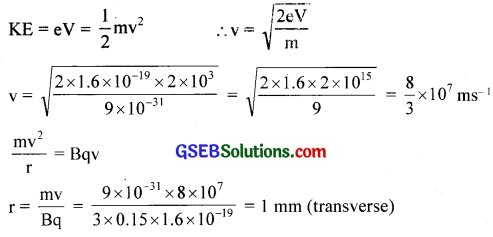(B) r = 0.5 mm inclined through 30° with B.
Here v sinθ component is r = 2.3r x 107ms-1

Question 19.
A magnetic field set up using Helmholtz coils (described in Exercise 15) is uniform in a small region and has a magnitude of 0.75 T. In the same region, a uniform electrostatic field is maintained in a direction normal to the common axis of the coils. A narrow beam of (single species) charged particles all accelerated through 15 kV enters this region in a direction perpendicular to both the axis of the coils and the electrostatic field. If the beam remains undeflected when the electrostatic field is 9.0 x 10+5 Vm-1, make a simple guess as to what the beam contains. Why is the answer not unique?
Solution:
Since the particle remains undeflected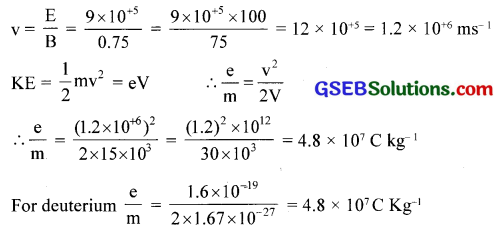Hence the particle is deuterium. Other particles are He, Li, etc.
The answer is not unique because only the ratio of the change to mass is determined.

Question 20.
A straight horizontal conducting rod of length 0.45 m and mass 60 g is suspended by two vertical wires at its ends. A current of 5.0 A is set up in the rod through the wires.
(a) What magnetic field should be set up normally to the conductor in order that the tension in the wires is zero?
(b) What will be the total tension in the wires if the direction of current is reversed keeping the magnetic field the same as before? (Ignore the mass of the wires.) g = 9.8 ms-2.
Solution:
I = 0.45 m and mass m = 60 g, I = 5A
(a) BIl = mg
∴ B = $$\frac{60 \times 10^{-3} \times 9.8 \times 100}{5 \times 0.45}$$

(b) 2 mg = 2 x 60 x 10-2 x 9.8 = 117.6 = 1.176 NQuestion 21.
The wires which connect the battery of an automobile to its starting motor carry a current of 300 A (for a short time). What is the force per unit length between the wires if they are 70 cm long and 1.5 cm apart? Is the force attractive or repulsive?
Solution:
I = 300 A, l = 70 cm, r = 1.5 cm
F = $$\frac{\mu_{0} I_{1} I_{2}}{2 \pi r}$$
= $$\frac{4 \pi \times 10^{-5} \times 300 \times 300}{2 \times 1.5 \times 10^{-2}}$$
= 1.2 N
The force is repulsive.

### GSEB Class 12 Physics Moving Charges and Magnetism Additional Important Questions and Answers

Question 1.
When current is passed through the conductor, the compass needle deflects. Why?
The compass needle is now subjected to the earth’s magnetic field and the magnetic field of the straight conductor.

Question 2.
Is the magnetic field the same at all points which are at the same distance from the element?
No. It depends on the sine of the angle between the current element and the radius vector, (i.e., dB ∝ sin θ).

Question 3.
Is the magnetic field influenced by the medium in which the conductor is placed?
Yes. The magnetic permeability modifies the field strength (dB ∝ μr)Question 4.
What is the field at any point outside the core of the solenoid?
Just at the outside on the axis, the field is half that well inside. F or a long solenoid, outside but close, the field is zero.

Question 5.
What is the field inside the core of the solenoid?
The field is uniform and is B = µ0nI where ‘n’ is the number of turns per unit length and I- the intensity of the current.

Question 6.
What is the nature of the field around a moving charge?
Its natural electric field and a magnetic field are due to its motional property.

Question 7.
Can a charge move in a magnetic field without experiencing a force?
If a charge moves parallel or antiparallel to an external field, then it does not experience a force.

Question 8.
What is the nature of the motion of charge entered inclined to a magnetic field?
Helical in path

Question 9.
If the path is circular, which force provides the necessary centripetal force?
The Lorentz magnetic forceQuestion 10.
What is the work done by magnetic force during this motion?
Zero (as displacement is perpendicular to force)

Question 11.
How can we confine the motion in a comparatively small space?
By forcing the charged particle to move along a circular or spiral path.

Question 12.
What is the electric field inside the ‘dees ’?
Inside the ‘dees’ electric field is zero.

Question 13.
What is the nature of the path inside the ‘dee ’?
Semicircular

Question 14.
The polarity of ‘dees’ should be changed when the charge completes the one-half circle. Why?
To accelerate the particle again in the electric field and is a phase with its motion.

Question 15.
“Torque is experienced by a current-carrying rectangular coil placed in a magnetic field.” Can this principle be used to detect feeble current?
Yes. Even if the current is feeble, torque is produced and deflection of the coil can be made notable.

Question 16.
What is the difference between a galvanometer and an ammeter?
The presence of current is detected using a galvanometer. The intensity or strength of the current is measured using an ammeter.Question 17.
Is it possible to measure high current using a galvanometer?
No. (It is sensitive to even small current)

Question 18.
How can you measure a high current using a galvanometer?
The galvanometer can be converted by connecting a shunt (or low) resistance across its terminals.

Question 19.
How will you connect a voltmeter to a circuit?
Parallel to the terminals across which voltage is to be read with proper polarity.

Question 20.
To measure p.d between two points in a circuit, the minimum current should pass through the voltmeter. Why?
Otherwise, the expected potential drop (as current x resistance) may not be produced.

Question 21.
How can you convert a galvanometer into an voltmeter?
By connecting a required high resistance in series with the galvanometer and suitably calibrat¬ing the dial.

Question 22.
What is the magnetic field at a point on the axial line on a current-carrying circular loop?
B = $$\frac{\mu_{0} I R^{2}}{2\left(R^{2}+x^{2}\right)^{3 / 2}}$$ (tesla)
where I – current, R – radius, x – distance from centre to the axial point.Question 23.
How can you rewrite the above equation in terms of area of the loop?
B = $$\frac{2 \mu_{0} I \cdot A}{4 \pi\left(R^{2}+x^{2}\right)^{3 / 2}}$$, where A = πR2 is the area.

Question 24.
An electric charge will experience a force in a uniform electric field. Similarly, a moving charge experiences a magnetic force (Lorentz) in a magnetic field. The SI unit of magnetic field intensity is defined in terms of Lorentz force.
(a) Write the expression for magnetic Lorentz force.
(b) Mention any two differences between electric and magnetic field.
(c) Give an account of work done by Lorentz force on a moving charge and corresponding change in K.E.
(a) $$\vec { F }$$ = q($$\vec { V }$$ x $$\vec { B }$$)

(b) Electric field is due to a charge, either in motion or at rest. The magnetic field is due to the motion of charge. Direction of electric force is collinear to electric field; direction of magnetic force is perpendicular to magnetic field.

(c) As Lorentz force is perpendicular to displacement (velocity), work done is zero and hence there is no change in K.E.Question 25.
Two infinitely long wires carry current as shown in Figure. Find the magnetic field intensity at the points P, P’ and P”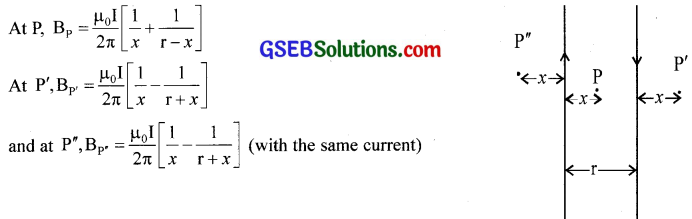Question 26.
Figure shows a long straight wire of finite cross-section carrying steady current i. The current i is uniformly distributed across this cross-section of radius a.
(i) Calculate the magnetic field in the region r < a and r > a.
(ii) Sketch the magnetic field against the distance from the axis of the wire.
(i) When r [For path I shown in fig, the current enclosed, i.e., $$\frac{\mathrm{i} \times \pi \mathrm{r}^{2}}{\pi \mathrm{a}^{2}}$$. Applying Ampere’s theorem, B.2πr = μ0$$\frac{\mathrm{ir}^{2}}{\mathrm{a}^{2}}$$ ∴ B = ( $$\frac{\mu_{0} \mathrm{i}}{2 \pi a^{2}}$$ )r ]
When r > a, B x 2πr = μ0i
∴ B = $$\frac{\mu_{0} \mathrm{i}}{2 \pi \mathrm{r}}$$

(ii)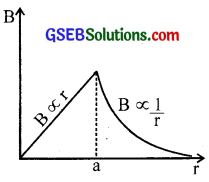Question 27.
A straight wire ABCD is bend as shown in Figure. Find an expression for the magnetic field at the point P?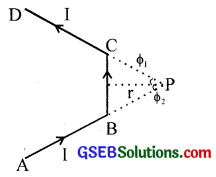Bp = $$\frac{\mu_{0} \mathrm{i}}{4 \pi \mathrm{r}}$$(sinΦ1 + sinΦ2
(No field on the axis of the straight conductor)

Question 28.
P and Q are two infinitely long straight parallel conductors placed in air ‘r ’ distance apart. Let I1 and I2, be the current on P and Q respectively flowing in the same direction. Then
(a) What is the magnetic field on Q due to If
(b) What is the force experienced by Q?
(c) Is there any attraction or repulsion between P and Q?
(a) Bp = $$\frac{\mu_{0} \mathrm{i}}{2 \pi \mathrm{r}}$$
(b) F = $$\frac{\mu_{0} I_{1} I_{2} l}{2 \pi r}$$ (over a length l)
(c) Yes. There is an attraction between P and Q.Question 29.
Describe qualitatively the path of a charged particle moving in a uniform magnetic field with an initial velocity
(a) Parallel to the field
(b) Perpendicular to the field and
(c) At an arbitrary angle with the field direction.
(a) Straight line
(b) Circular path
(c) Helix

Question 30.
When a charged particle moves in a magnetic field, does its KE always remain constant? Explain.
A charged particle moving in a magnetic field of constant intensity does not gain kinetic energy. This is because the force is $$\vec { F }$$ = q(v$$\vec { v }$$ x $$\vec { B }$$) and work done, W = $$\vec { f }$$.$$\vec { s }$$ or in 1 second, $$\vec { F }$$.$$\vec { v }$$ = q($$\vec { v }$$ x $$\vec { B }$$)$$\vec { v }$$ = 0 because of property of scalar triple product.

Question 31.
A long copper wire of diameter 2 mm carries a current of 1.5 A.
(a) What is the magnetic field (B) at a perpendicular distance 1 m from the middle of the wire?
(b) What is the magnetic field at a perpendicular distance 1 m from one end of the wire?
(c) What is the field (B) on the surface of the conductor?
(d) At a distance 1 mm from the axis, what is the value of the magnetic field?
Data supplied,
Radius r = 1 mm = 1 x 10-3m, I = 1.5 A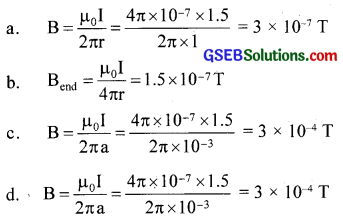Question 32.
An electron does not suffer any deflection while passing through a region Are you sure that there is no magnetic field?
No, because the electron suffers no force when it moves parallel or antiparallel to the magnetic field. So the magnetic field may exist parallel or antiparallel to the motion of electron.Question 33.
On looking downward, an electron appears moving anti-clockwise in a horizontal circle under a magnetic field. What is the direction of the field?
Vertically upward

Question 34.
If a thin wire of length 88 cm is bent into a circular loop and a current of 1A is passed,
(a) What is the magnetic field at the centre?
(b) What is the magnetic field at the centre if the wire is bent into a semicircle and doubled on it?
(c) If the wire is looped into two loops, find the magnetic field at the centre.
(a)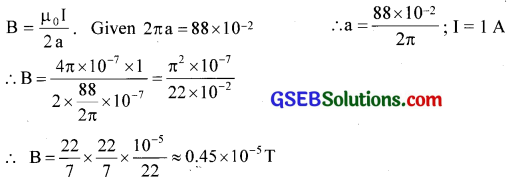(b) Zero
(c) ≈ 1.8 x 10-5T

Question 35.
A current carrying a circular loop lies on a smooth horizontal plane. Can a uniform magnetic field be set up in such a manner that the loop turns around itself?
No, the torque on a current loop is given by $$\vec { τ }$$ = $$\vec { M }$$ x $$\vec { B }$$ = I$$\vec { A}$$ x $$\vec { B }$$
The loop can turn around itself only when f is along vertical. Since A is vertical, x cannot be vertical.

Question 36.
A uniform magnetic field of 3000G is established along with the positive $$\vec { τ }$$ – direction. A rectangular loop of sides 10 cm and 5 cm carries a current of 12A. What is the torque on the loop in different cases shown in the figure? What is the force on each case? Which case corresponds to stable equilibrium?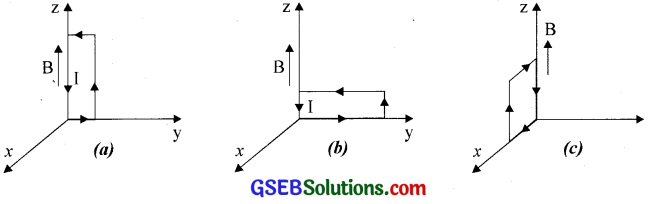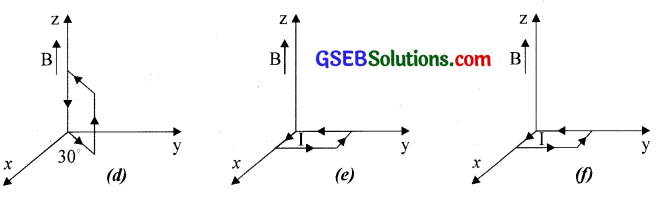1. τ = I($$\vec { A}$$ x $$\vec { B }$$)
In fig. a. 1.8 x 10-2 Nm along – y direction
In fig. b. same as in (a)
In fig. c. 1.8 x 10-2 Nm along – x direction.
In fig. d. 1.8 x 10-2 Nm at an angle of 240° along the x direction. In e and f the torque is zero.

2. Force is zero in each case.
Fig. e. corresponds to stable equilibrium.

Question 37.
Describe qualitatively the path of a charged particle moving in
(a) a uniform electrostatic field, with initial velocity (i) parallel to the field, (ii) perpendicular to the field, (iii) at an arbitrary angle with the field direction.
(b) a uniform magnetic field, with initial velocity (i) parallel to the field, (ii) perpendicular to the field, (iii) at an arbitrary angle with the field direction.
(a) Force on the charge, F = qE.
i. The speed increases if q is positive, otherwise decreases
ii. Initial velocity remains constant and in the field it is accelerated.
iii. The initial velocity may be resolved. The perpendicular component remains unaffected.

(b) i. No change for direction and velocity.
ii. The path becomes circular.
iii. Generally, helix.Question 38.
A uniform magnetic field of 1.5 T exists in a cylindrical region of radius 10.0 cm, its direction parallel to the axis is along east to west. A wire carrying current of 7.0 A in the north to south direction passes through this region. What is the magnitude and direction of the force on the wire if,
(a) the wire intersects the axis,
(b) the wire is turned from N-S to north east-north west direction,
(c) the wire in the N-S direction is lowered from the axis by a distance of 6.0 cm?
(a) 2.1 N vertically downwards
(b) 2.1 N vertically downwards (remember / sin 0 is constant)
(c) 1.68 N vertically downwards.

Question 39.
A straight horizontal conducting rod of length 0.45 m and mass 60 g is suspended by two vertical wires at its ends. A current of 5.0 A is set up in the rod through the wires.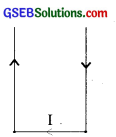(a) What magnetic field should be set up normal to the conductor in order that the tension in the wires is zero?
(b) What will be the total tension in the wires if the direction of current is reversed keeping the magnetic field the same as before? (Ignore the mass of the wires.) g = 9.8 m s-2.
(a) BIl = mg, B = 0.26 T

(b) 2 mg = 1.176 N

Question 40.
A current carrying loop of irregular shape is located in an external magnetic field. If the wire is flexible, why does it become circular?
For a given perimeter, a circle encloses greatest area than any other shape. Thus in order to involve the maximum number of field lines, the coil assumes a circular shape with its plane normal to the field lines.

Question 41.
Starting from the expression for the force on a current-carrying conductor in a magnetic field, arrive at the expression for Lorentz magnetic force.
Force = BIl = B x $$\frac { q }{ t }$$l But $$\frac { l }{ t }$$ = v
∴ F = Bqv or $$\bar { F }$$ = q($$\bar { v }$$ x $$\bar { B }$$) – the Lorentz magnetic force.

Question 42.
(a) What type of particles can be accelerated in a cyclotron?
(b) Why cyclotron is not used to accelerate electrons and neutrons?
(a) Proton, deutron. α – particle etc.
(b) As the electron gets accelerated, its frequency of revolution changes from that of the applied electric field. Neutron is uncharged.Question 43.
In a moving coil galvanometer,
(a) why is a horse-shoe magnet used?
(b) why is phosphor bronze fiber used?
(c) why is a soft iron cylinder used?
(a) To produce a cylindrical field with radial lines.
(b) Phosphor bronze fibre is a good elastic material with a small couple per unit twist.
(c) To concentrate the lines of force so that the field is uniform in the region in which the coil is placed.

Question 44.
Expression for voltage sensitivity is $$\frac { θ }{ V }$$ = $$\frac { NAB }{ CR }$$
(a) What are the factors on which voltage sensitivity depends?
(b) Why is it independent of number of turns?
(c) How will you increase the voltage sensitivity?
(a) i. $$\frac { N }{ R }$$
ii. A
iii. B
iv. C

(b) $$\frac { N }{ R }$$ is a constant.

(c) Voltage sensitivity can be increased by
i. increasing magnetic field (B)
ii. increasing area (A)
iii. decreasing torque per unit twist (C)

Question 45.
A moving coil galvanometer can be converted into an ammeter.
(a) Is the statement true or false?
(b) If true, how is it possible? Explain
(c) What is the effective resistance?
(a) True
(b) By connecting a suitable low resistance (short) parallel to it.
(c) Less than that of the shunt.

Question 46.
No net force acts on a rectangular loop carrying a steady current when suspended freely in a uniform magnetic field. Is the statement correct or wrong? Justify your answer.
Correct. The forces on pair of opposite sides are equal and opposite hence no net force.

Question 47.
A student records the following data for the magnitudes (B) of the magnetic field at axial points at different distances x from the centre of a circular coil of radius ‘a ’ carrying a current 7’. Verify (for any two) that these observations are in good agreement with the expected theoretical variation of B with x.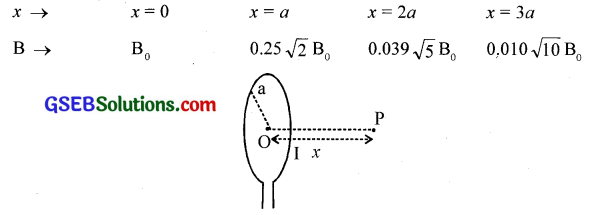Magnetic field (B) is given by; B = $$\frac { { \mu }_{ 0 } }{ 4\pi }$$$$\frac{2 \mathrm{M}}{x^{3}}$$
It is evident that as x increases, the value of B decreases which is shown by the observations also.

Question 48.
What is the magnitude of the induced current in the circular loop KLMN of radius ‘r’ if the straight wire PQ carries a steady current of magnitude ‘i’ ampere?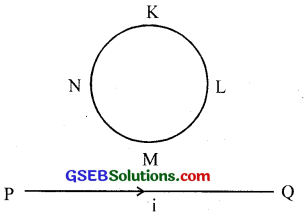Zero

Question 49.
A short bar magnet placed with its axis at 30° to a uniform magnetic field of 0.2 T experiences a torque of 0.060 Nm.
(i) Calculate magnetic moment of the magnet
(ii) Find out what orientation of the magnet corresponds to its stable equilibrium in the magnetic field.
(i) Here τ = 0.060 Nm, θ = 30°, B = 0.2 T
As τ = mB sin θ
∴ 0.060 = m x 0.2 x sin 30°
∴ m = $$\frac { 0.060 }{ 0.2×0.5 }$$
= 0.06 JT-1

(ii) PE of magnet in field B is given by
U = -mBcosθ
When magnet is placed parallel to $$\vec { B }$$, θ = 0° and
U = -mB cos 0° = -mB
i.e., the PE of the dipole is minimum. This is the state of stable equilibrium.Question 50.
In the diagram shown is a circular loop carrying current I. Show the direction of the magnetic field with the help of lines of force.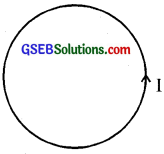The magnetic lines of force of a circular loop carrying current I are shown below.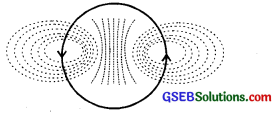Question 51.
Two long parallel straight wires X and Y separated by a distance of 5 cm in the air carry currents of 10A and 5A respectively in opposite directions. Calculate the magnitude and direction of the force on a 20 cm length of the wire Y.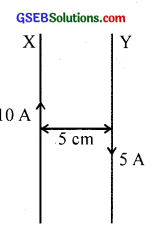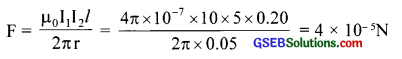The direction of the force is perpendicular to the length of wire Y and acts away from X (repulsion).

Question 52.
A galvanometer with a coil of resistance 120 ohm shows full-scale deflection for a current of 2.5 mA. How will you convert the galvanometer into an ammeter of range 0 to 7.5A? Determine the net resistance of the ammeter. When the ammeter is put in a circuit, does it read slightly less or more than the actual current in the original circuit? Justify your answer.
Rg = 120Ω. Ig = 2.5 mA=0.0025A, 1 = 7.5 A
Rs = $$\frac{\mathrm{I}_{\mathrm{g}}}{\mathrm{I}-\mathrm{I}_{\mathrm{g}}}$$ x Rg
= $$\frac { 0.0025 }{ 7.5-0.0025 }$$ x 120
= 0.04 Ω
By connecting a shunt of 0.04Ω across the given galvanometer, we get an ammeter of range 0 to 7.5 A.
Net resistance of the ammeter = $$\frac { 120 × 0.04 }{ 120+0.04 }$$ = 0.03998 Ω
When an ammeter is put in a circuit, it reads slightly less than the actual current. An ammeter has a small resistance. When it is connected to the circuit, it decreases the current by a small amount.Question 53.
Two wires of equal lengths are bent in the form of two loops. One of the loops is square-shaped whereas the other loop is circular. These are suspended in a uniform magnetic field and the same current is passed through them. Which loop will experience greater torque? Give reason.